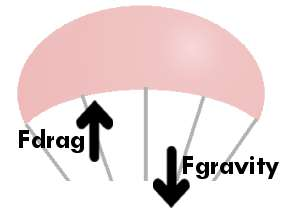## Math and Theory

### How does a parachute work?A parachute works by increasing the surface area of an object so it has more contact with the wind, which decreases the speed of the object. In terms of rocketry, a rocket will hit the ground at about the same speed that it is launched off with. You might not think that you need a parachute, but in reality if you don’t have a parachute you could seriously damage an important part of your rocket which would render it useless.

### Isn’t bigger better?

You can’t make an enormous parachute and ignore the math. It won’t work for two reasons. First, you won’t have enough room in your rocket for a super huge chute. Second, the bigger your chute, the longer your rocket will be in the air, and the longer the wind will have to push your rocket away from where you want it to land. Ideally you want your parachute to be big enough so your rocket has a soft landing but small enough that the rocket returns to the ground in a timely manner.

### How big should it be?

Well, there’s two formulas that we’ll use. Basically we’re setting the force of gravity equal to the force of the wind pushing us up. I’m about to show you how to derive the final master equation, but if you don’t care just scroll down and the final equation is at the bottom of the page. Here’s our two equations:

Fdrag = 1/2 * Density * Cd * Area * Velocity2

Fgravity = mass * gravity

Fdrag is the force of the wind pushing the rocket upward (in Newtons), Density is the density of air (1.22 kg/m^3), Cd is the drag coefficient (.75 if you have a flat shape, 1.5 if you have a dome shape), Area is the total surface area (in m2), and finally Velocity is the speed at which the rocket will be traveling when it hits the ground (in m/s2). In the second equation, Fgravity is the force that gravity puts on the rocket (in Newtons), mass is the weight of the entire rocket (in kilograms), and gravity is the acceleration of gravity (9.8 m/s2).

### Purpose of the Equations

Like I said earlier — we’re just going to set them equal to each other and solve for the area (that way, we can determine how big to make our canopy). Here’s the math to solve for area:

½ * D * Cd * A * V2 = m * g

A = m * g * 2 / (D * Cd * V2)

(however, I’m making a circular canopy…so replace area using area = pi * r2)

pi * r2 = m * g * 2 / (D * Cd * V2)

r = square root of [ m * g * 2 / (D * Cd * pi * V2) ]

(let’s solve for diameter — diameter = 2 * radius)

d = 2 * square root of [ m * g * 2 / (D * Cd * pi * V2) ]

d = square root of [ 8 * m * g / (D * Cd * pi * V2) ]

Assuming we’re on earth, we can plug in density of air, pi, and gravity…

d = 4.52 * square root of [ m / (Cd * V2) ]

For those who didn’t care about how to get the equations and just wanted to scroll down, here you go. The top equation is an equation if you’re doing a flat parachute (which already has a Cd of .75 plugged into it) and the bottom equation is an equation if you’re doing a dome-style parachute (which already has a Cd of 1.5 plugged into it). Remember: velocity is in m/s and mass is in kilograms!

surface area = 21.4 * mass / velocity2

diameter = 3.69 * square root of [ mass / velocity2 ]

If you’re feeling especially lazy, just use this calculator and it’ll figure out your area or diameter for you…

 Parachute Calculator Flat chute Dome-style Mass (in kilograms) Final velocity (in m/s)

Next: My Calculations